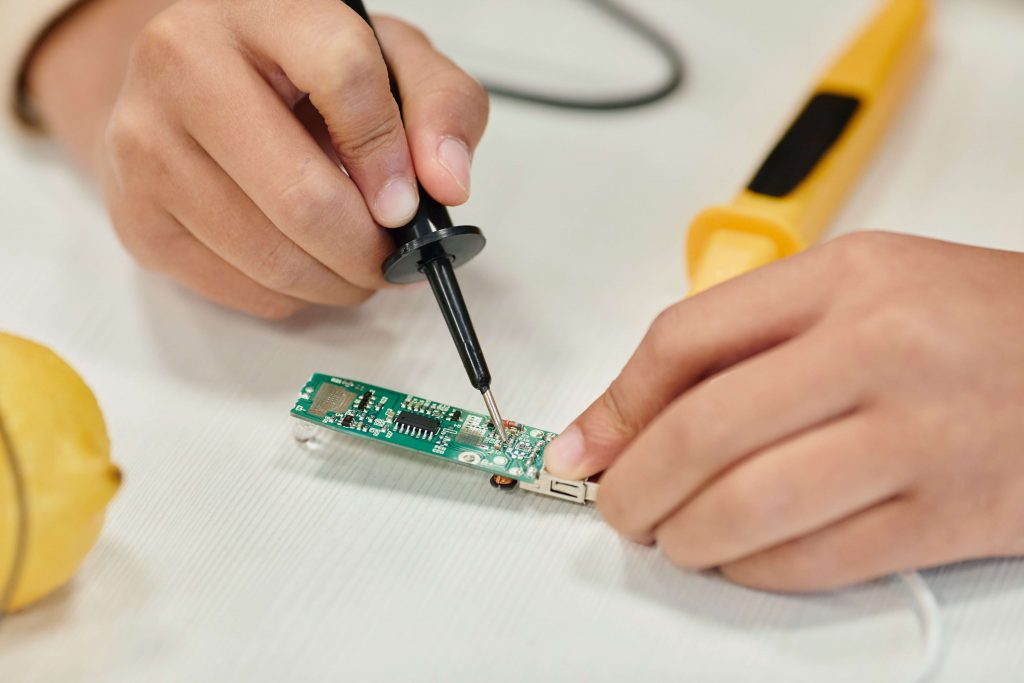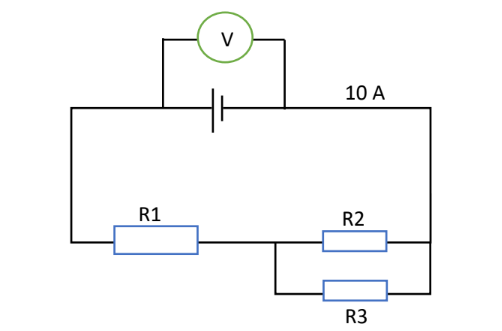# Sec 2 Science Core Topic – Electricity

## 19 Jul Sec 2 Science Core Topic – ElectricityWhy is electricity an important topic?

Electricity is a core topic in lower secondary Science. As a topic first taught in Sec 2, it introduces important concepts to the students for their upper secondary physics. Students are required to have a sound knowledge and apply the major concepts in electricity to enhance their understanding of Combined or Pure Physics in Sec 3 and Sec 4. Moreover, students, who aim to enrol in Science, Engineering and IT courses, will be required to study electricity as a compulsory topic in their tertiary education. The knowledge and skills that they have acquired in lower secondary electricity topic will serve as a strong foundation for their upcoming future studies and beyond, their career.

Critical Concepts

Students will learn the symbols of the electrical components and how to draw the basic electrical circuit diagram correctly. Methods of connecting an ammeter and voltmeter to the electrical components in the circuit will also be taught as well.

In terms of calculation, students will apply the formulas to calculate the total resistance of resistors in parallel and series arrangement.

Furthermore, the famous Ohm’s law, an essential yet very fundamental concept, is introduced to the students in the topic of electricity in Sec 2.  Using this important concept, they will appreciate the relationship between potential difference and resistance, as well as how they affect electric current.

ExampleGiven that R1 = 4.5 Ω, R2=R3= 1 Ω, find the potential difference value shown on the voltmeter.

Explanation: In order to find the reading at the voltmeter, student needs to find the total resistance of the entire circuit first. Once they have found the total resistance, they should be able to use Ohm’s law formula to find the potential difference.

Solution: R2 and R3 are in parallel arrangement.

1/R2+3 = 1/R2 + 1/R3

1/R2+3 = 1/1 + 1/1

1/R2+3 = 2/1

After cross-multiply,

1 = 2 R2+3

R2+3 = 1/2 Ω= 0.5 Ω

R1 and R2+3 are in series arrangement

Therefore, total Resistance, R = R1 + R2+3  =  4.5 Ω + 0.5 Ω = 5 Ω

Using Ohm’s Law, R = V/I

V = IR = 10 x 5 = 50 V

How to excel in the topic?

The electricity topic requires the students not only to remember the concepts correctly, but also understand the linkage between the three key components in current, resistance, and potential difference.

First of all, students need to understand the definition and meaning of each key component (current, resistance, and potential difference); how these components affect each other in parallel and series arrangement; and how an ammeter and voltmeter can be connected in a circuit. Understanding those fundamentals will help the students to retain the concepts over a longer period of time without causing confusion. This in turn raises their accuracy when answering the basic MCQs and structured questions.

Secondly, students must be proficient enough to understand how resistors are arranged in a circuit diagram, either in a parallel or series arrangement. Students who master reading circuit diagrams will have no difficulty in calculating the total resistance of the whole circuit.

Lastly, students will be required to apply the Ohm’s law competently to calculate the potential difference, electrical current or resistance within a circuit. Application is a higher-order learning objective. Therefore, a deeper understanding and correct application will enable the students to answer challenging MCQs and structured questions more confidently.

Einstein Takeaway

There are many important and fundamental concepts that students need to master in lower secondary Science. Einstein Achievers’ tuition classes train up the students to deliver clear and in-depth explanations on the Physics, Biology and Chemistry topics in Sec 1 and Sec 2.  Contact Einstein now to gain useful insights on the effective critical thinking skills that the students successfully develop at Einstein.